# tf.data: Build TensorFlow input pipelines

Stay organized with collections Save and categorize content based on your preferences.

The `tf.data` API enables you to build complex input pipelines from simple, reusable pieces. For example, the pipeline for an image model might aggregate data from files in a distributed file system, apply random perturbations to each image, and merge randomly selected images into a batch for training. The pipeline for a text model might involve extracting symbols from raw text data, converting them to embedding identifiers with a lookup table, and batching together sequences of different lengths. The `tf.data` API makes it possible to handle large amounts of data, read from different data formats, and perform complex transformations.

The `tf.data` API introduces a `tf.data.Dataset` abstraction that represents a sequence of elements, in which each element consists of one or more components. For example, in an image pipeline, an element might be a single training example, with a pair of tensor components representing the image and its label.

There are two distinct ways to create a dataset:

• A data source constructs a `Dataset` from data stored in memory or in one or more files.

• A data transformation constructs a dataset from one or more `tf.data.Dataset` objects.

``````import tensorflow as tf
``````
```2022-12-14 02:25:11.285549: W tensorflow/compiler/xla/stream_executor/platform/default/dso_loader.cc:64] Could not load dynamic library 'libnvinfer.so.7'; dlerror: libnvinfer.so.7: cannot open shared object file: No such file or directory
2022-12-14 02:25:11.285658: W tensorflow/compiler/xla/stream_executor/platform/default/dso_loader.cc:64] Could not load dynamic library 'libnvinfer_plugin.so.7'; dlerror: libnvinfer_plugin.so.7: cannot open shared object file: No such file or directory
2022-12-14 02:25:11.285669: W tensorflow/compiler/tf2tensorrt/utils/py_utils.cc:38] TF-TRT Warning: Cannot dlopen some TensorRT libraries. If you would like to use Nvidia GPU with TensorRT, please make sure the missing libraries mentioned above are installed properly.
```
``````import pathlib
import os
import matplotlib.pyplot as plt
import pandas as pd
import numpy as np

np.set_printoptions(precision=4)
``````

## Basic mechanics

To create an input pipeline, you must start with a data source. For example, to construct a `Dataset` from data in memory, you can use `tf.data.Dataset.from_tensors()` or `tf.data.Dataset.from_tensor_slices()`. Alternatively, if your input data is stored in a file in the recommended TFRecord format, you can use `tf.data.TFRecordDataset()`.

Once you have a `Dataset` object, you can transform it into a new `Dataset` by chaining method calls on the `tf.data.Dataset` object. For example, you can apply per-element transformations such as `Dataset.map`, and multi-element transformations such as `Dataset.batch`. Refer to the documentation for `tf.data.Dataset` for a complete list of transformations.

The `Dataset` object is a Python iterable. This makes it possible to consume its elements using a for loop:

``````dataset = tf.data.Dataset.from_tensor_slices([8, 3, 0, 8, 2, 1])
dataset
``````
```<TensorSliceDataset element_spec=TensorSpec(shape=(), dtype=tf.int32, name=None)>
```
``````for elem in dataset:
print(elem.numpy())
``````
```8
3
0
8
2
1
```

Or by explicitly creating a Python iterator using `iter` and consuming its elements using `next`:

``````it = iter(dataset)

print(next(it).numpy())
``````
```8
```

Alternatively, dataset elements can be consumed using the `reduce` transformation, which reduces all elements to produce a single result. The following example illustrates how to use the `reduce` transformation to compute the sum of a dataset of integers.

``````print(dataset.reduce(0, lambda state, value: state + value).numpy())
``````
```WARNING:tensorflow:From /tmpfs/src/tf_docs_env/lib/python3.9/site-packages/tensorflow/python/autograph/pyct/static_analysis/liveness.py:83: Analyzer.lamba_check (from tensorflow.python.autograph.pyct.static_analysis.liveness) is deprecated and will be removed after 2023-09-23.
Instructions for updating:
Lambda fuctions will be no more assumed to be used in the statement where they are used, or at least in the same block. https://github.com/tensorflow/tensorflow/issues/56089
22
```

### Dataset structure

A dataset produces a sequence of elements, where each element is the same (nested) structure of components. Individual components of the structure can be of any type representable by `tf.TypeSpec`, including `tf.Tensor`, `tf.sparse.SparseTensor`, `tf.RaggedTensor`, `tf.TensorArray`, or `tf.data.Dataset`.

The Python constructs that can be used to express the (nested) structure of elements include `tuple`, `dict`, `NamedTuple`, and `OrderedDict`. In particular, `list` is not a valid construct for expressing the structure of dataset elements. This is because early `tf.data` users felt strongly about `list` inputs (for example, when passed to `tf.data.Dataset.from_tensors`) being automatically packed as tensors and `list` outputs (for example, return values of user-defined functions) being coerced into a `tuple`. As a consequence, if you would like a `list` input to be treated as a structure, you need to convert it into `tuple` and if you would like a `list` output to be a single component, then you need to explicitly pack it using `tf.stack`.

The `Dataset.element_spec` property allows you to inspect the type of each element component. The property returns a nested structure of `tf.TypeSpec` objects, matching the structure of the element, which may be a single component, a tuple of components, or a nested tuple of components. For example:

``````dataset1 = tf.data.Dataset.from_tensor_slices(tf.random.uniform([4, 10]))

dataset1.element_spec
``````
```TensorSpec(shape=(10,), dtype=tf.float32, name=None)
```
``````dataset2 = tf.data.Dataset.from_tensor_slices(
(tf.random.uniform(),
tf.random.uniform([4, 100], maxval=100, dtype=tf.int32)))

dataset2.element_spec
``````
```(TensorSpec(shape=(), dtype=tf.float32, name=None),
TensorSpec(shape=(100,), dtype=tf.int32, name=None))
```
``````dataset3 = tf.data.Dataset.zip((dataset1, dataset2))

dataset3.element_spec
``````
```(TensorSpec(shape=(10,), dtype=tf.float32, name=None),
(TensorSpec(shape=(), dtype=tf.float32, name=None),
TensorSpec(shape=(100,), dtype=tf.int32, name=None)))
```
``````# Dataset containing a sparse tensor.
dataset4 = tf.data.Dataset.from_tensors(tf.SparseTensor(indices=[[0, 0], [1, 2]], values=[1, 2], dense_shape=[3, 4]))

dataset4.element_spec
``````
```SparseTensorSpec(TensorShape([3, 4]), tf.int32)
```
``````# Use value_type to see the type of value represented by the element spec
dataset4.element_spec.value_type
``````
```tensorflow.python.framework.sparse_tensor.SparseTensor
```

The `Dataset` transformations support datasets of any structure. When using the `Dataset.map`, and `Dataset.filter` transformations, which apply a function to each element, the element structure determines the arguments of the function:

``````dataset1 = tf.data.Dataset.from_tensor_slices(
tf.random.uniform([4, 10], minval=1, maxval=10, dtype=tf.int32))

dataset1
``````
```<TensorSliceDataset element_spec=TensorSpec(shape=(10,), dtype=tf.int32, name=None)>
```
``````for z in dataset1:
print(z.numpy())
``````
```[1 6 9 2 8 7 1 9 2 4]
[2 1 5 2 6 9 1 4 5 3]
[8 5 6 6 7 4 5 1 8 4]
[8 5 8 1 9 3 9 2 9 3]
```
``````dataset2 = tf.data.Dataset.from_tensor_slices(
(tf.random.uniform(),
tf.random.uniform([4, 100], maxval=100, dtype=tf.int32)))

dataset2
``````
```<TensorSliceDataset element_spec=(TensorSpec(shape=(), dtype=tf.float32, name=None), TensorSpec(shape=(100,), dtype=tf.int32, name=None))>
```
``````dataset3 = tf.data.Dataset.zip((dataset1, dataset2))

dataset3
``````
```<ZipDataset element_spec=(TensorSpec(shape=(10,), dtype=tf.int32, name=None), (TensorSpec(shape=(), dtype=tf.float32, name=None), TensorSpec(shape=(100,), dtype=tf.int32, name=None)))>
```
``````for a, (b,c) in dataset3:
print('shapes: {a.shape}, {b.shape}, {c.shape}'.format(a=a, b=b, c=c))
``````
```shapes: (10,), (), (100,)
shapes: (10,), (), (100,)
shapes: (10,), (), (100,)
shapes: (10,), (), (100,)
```

### Consuming NumPy arrays

If all of your input data fits in memory, the simplest way to create a `Dataset` from them is to convert them to `tf.Tensor` objects and use `Dataset.from_tensor_slices`.

``````train, test = tf.keras.datasets.fashion_mnist.load_data()
``````
```Downloading data from https://storage.googleapis.com/tensorflow/tf-keras-datasets/train-labels-idx1-ubyte.gz
29515/29515 [==============================] - 0s 0us/step
26421880/26421880 [==============================] - 0s 0us/step
5148/5148 [==============================] - 0s 0us/step
4422102/4422102 [==============================] - 0s 0us/step
```
``````images, labels = train
images = images/255

dataset = tf.data.Dataset.from_tensor_slices((images, labels))
dataset
``````
```<TensorSliceDataset element_spec=(TensorSpec(shape=(28, 28), dtype=tf.float64, name=None), TensorSpec(shape=(), dtype=tf.uint8, name=None))>
```

### Consuming Python generators

Another common data source that can easily be ingested as a `tf.data.Dataset` is the python generator.

``````def count(stop):
i = 0
while i<stop:
yield i
i += 1
``````
``````for n in count(5):
print(n)
``````
```0
1
2
3
4
```

The `Dataset.from_generator` constructor converts the python generator to a fully functional `tf.data.Dataset`.

The constructor takes a callable as input, not an iterator. This allows it to restart the generator when it reaches the end. It takes an optional `args` argument, which is passed as the callable's arguments.

The `output_types` argument is required because `tf.data` builds a `tf.Graph` internally, and graph edges require a `tf.dtype`.

``````ds_counter = tf.data.Dataset.from_generator(count, args=, output_types=tf.int32, output_shapes = (), )
``````
``````for count_batch in ds_counter.repeat().batch(10).take(10):
print(count_batch.numpy())
``````
```[0 1 2 3 4 5 6 7 8 9]
[10 11 12 13 14 15 16 17 18 19]
[20 21 22 23 24  0  1  2  3  4]
[ 5  6  7  8  9 10 11 12 13 14]
[15 16 17 18 19 20 21 22 23 24]
[0 1 2 3 4 5 6 7 8 9]
[10 11 12 13 14 15 16 17 18 19]
[20 21 22 23 24  0  1  2  3  4]
[ 5  6  7  8  9 10 11 12 13 14]
[15 16 17 18 19 20 21 22 23 24]
```

The `output_shapes` argument is not required but is highly recommended as many TensorFlow operations do not support tensors with an unknown rank. If the length of a particular axis is unknown or variable, set it as `None` in the `output_shapes`.

It's also important to note that the `output_shapes` and `output_types` follow the same nesting rules as other dataset methods.

Here is an example generator that demonstrates both aspects: it returns tuples of arrays, where the second array is a vector with unknown length.

``````def gen_series():
i = 0
while True:
size = np.random.randint(0, 10)
yield i, np.random.normal(size=(size,))
i += 1
``````
``````for i, series in gen_series():
print(i, ":", str(series))
if i > 5:
break
``````
```0 : [0.2686 0.3138]
1 : [ 0.2136  1.8005 -1.9186 -0.8836  0.1731 -0.0278 -0.2359  0.7901]
2 : [ 0.5355 -1.646   1.1176  0.5501 -1.6814 -0.2788 -2.7651  0.0617  0.087 ]
3 : [-0.1393 -1.5706 -0.576  -0.9833]
4 : [-0.5383 -1.4567 -0.2296  0.6741 -2.0608]
5 : [ 0.0842 -1.7841  0.4571 -0.482   1.0696]
6 : [-1.5967 -0.0396]
```

The first output is an `int32` the second is a `float32`.

The first item is a scalar, shape `()`, and the second is a vector of unknown length, shape `(None,)`

``````ds_series = tf.data.Dataset.from_generator(
gen_series,
output_types=(tf.int32, tf.float32),
output_shapes=((), (None,)))

ds_series
``````
```<FlatMapDataset element_spec=(TensorSpec(shape=(), dtype=tf.int32, name=None), TensorSpec(shape=(None,), dtype=tf.float32, name=None))>
```

Now it can be used like a regular `tf.data.Dataset`. Note that when batching a dataset with a variable shape, you need to use `Dataset.padded_batch`.

``````ds_series_batch = ds_series.shuffle(20).padded_batch(10)

ids, sequence_batch = next(iter(ds_series_batch))
print(ids.numpy())
print()
print(sequence_batch.numpy())
``````
```[12  1 19  9  8 24 13 23  3  4]

[[ 1.038  -0.4861  1.2788 -0.5632  0.0042  0.      0.      0.    ]
[-0.1249 -2.0747  0.0953 -1.3645 -0.8358  1.0512 -1.6868  0.1321]
[ 0.316   0.637   0.6635  1.0732  0.      0.      0.      0.    ]
[ 0.2399 -2.464   0.      0.      0.      0.      0.      0.    ]
[ 0.      0.      0.      0.      0.      0.      0.      0.    ]
[ 0.      0.      0.      0.      0.      0.      0.      0.    ]
[ 0.9888  0.3011 -0.8368 -1.4325  0.4583  2.2669 -0.9532  0.    ]
[-1.2344 -1.5927  0.5593  0.      0.      0.      0.      0.    ]
[-0.9541  0.314  -1.4863 -2.4093  0.8278  0.8515 -2.1328  0.    ]
[-2.8132 -0.0401 -0.9833 -2.5067 -0.0451  0.      0.      0.    ]]
```

For a more realistic example, try wrapping `preprocessing.image.ImageDataGenerator` as a `tf.data.Dataset`.

``````flowers = tf.keras.utils.get_file(
'flower_photos',
untar=True)
``````
```Downloading data from https://storage.googleapis.com/download.tensorflow.org/example_images/flower_photos.tgz
228813984/228813984 [==============================] - 1s 0us/step
```

Create the `image.ImageDataGenerator`

``````img_gen = tf.keras.preprocessing.image.ImageDataGenerator(rescale=1./255, rotation_range=20)
``````
``````images, labels = next(img_gen.flow_from_directory(flowers))
``````
```Found 3670 images belonging to 5 classes.
```
``````print(images.dtype, images.shape)
print(labels.dtype, labels.shape)
``````
```float32 (32, 256, 256, 3)
float32 (32, 5)
```
``````ds = tf.data.Dataset.from_generator(
lambda: img_gen.flow_from_directory(flowers),
output_types=(tf.float32, tf.float32),
output_shapes=([32,256,256,3], [32,5])
)

ds.element_spec
``````
```(TensorSpec(shape=(32, 256, 256, 3), dtype=tf.float32, name=None),
TensorSpec(shape=(32, 5), dtype=tf.float32, name=None))
```
``````for images, labels in ds.take(1):
print('images.shape: ', images.shape)
print('labels.shape: ', labels.shape)
``````
```Found 3670 images belonging to 5 classes.
images.shape:  (32, 256, 256, 3)
labels.shape:  (32, 5)
```

### Consuming TFRecord data

The `tf.data` API supports a variety of file formats so that you can process large datasets that do not fit in memory. For example, the TFRecord file format is a simple record-oriented binary format that many TensorFlow applications use for training data. The `tf.data.TFRecordDataset` class enables you to stream over the contents of one or more TFRecord files as part of an input pipeline.

Here is an example using the test file from the French Street Name Signs (FSNS).

``````# Creates a dataset that reads all of the examples from two files.
``````
```Downloading data from https://storage.googleapis.com/download.tensorflow.org/data/fsns-20160927/testdata/fsns-00000-of-00001
7904079/7904079 [==============================] - 0s 0us/step
```

The `filenames` argument to the `TFRecordDataset` initializer can either be a string, a list of strings, or a `tf.Tensor` of strings. Therefore if you have two sets of files for training and validation purposes, you can create a factory method that produces the dataset, taking filenames as an input argument:

``````dataset = tf.data.TFRecordDataset(filenames = [fsns_test_file])
dataset
``````
```<TFRecordDatasetV2 element_spec=TensorSpec(shape=(), dtype=tf.string, name=None)>
```

Many TensorFlow projects use serialized `tf.train.Example` records in their TFRecord files. These need to be decoded before they can be inspected:

``````raw_example = next(iter(dataset))
parsed = tf.train.Example.FromString(raw_example.numpy())

parsed.features.feature['image/text']
``````
```bytes_list {
value: "Rue Perreyon"
}
```

### Consuming text data

Refer to the Load text tutorial for an end-to-end example.

Many datasets are distributed as one or more text files. The `tf.data.TextLineDataset` provides an easy way to extract lines from one or more text files. Given one or more filenames, a `TextLineDataset` will produce one string-valued element per line of those files.

``````directory_url = 'https://storage.googleapis.com/download.tensorflow.org/data/illiad/'
file_names = ['cowper.txt', 'derby.txt', 'butler.txt']

file_paths = [
tf.keras.utils.get_file(file_name, directory_url + file_name)
for file_name in file_names
]
``````
```Downloading data from https://storage.googleapis.com/download.tensorflow.org/data/illiad/cowper.txt
815980/815980 [==============================] - 0s 0us/step
809730/809730 [==============================] - 0s 0us/step
807992/807992 [==============================] - 0s 0us/step
```
``````dataset = tf.data.TextLineDataset(file_paths)
``````

Here are the first few lines of the first file:

``````for line in dataset.take(5):
print(line.numpy())
``````
```b"\xef\xbb\xbfAchilles sing, O Goddess! Peleus' son;"
b'His wrath pernicious, who ten thousand woes'
b"Caused to Achaia's host, sent many a soul"
b'And Heroes gave (so stood the will of Jove)'
```

To alternate lines between files use `Dataset.interleave`. This makes it easier to shuffle files together. Here are the first, second and third lines from each translation:

``````files_ds = tf.data.Dataset.from_tensor_slices(file_paths)
lines_ds = files_ds.interleave(tf.data.TextLineDataset, cycle_length=3)

for i, line in enumerate(lines_ds.take(9)):
if i % 3 == 0:
print()
print(line.numpy())
``````
```b"\xef\xbb\xbfAchilles sing, O Goddess! Peleus' son;"
b"\xef\xbb\xbfOf Peleus' son, Achilles, sing, O Muse,"
b'\xef\xbb\xbfSing, O goddess, the anger of Achilles son of Peleus, that brought'

b'His wrath pernicious, who ten thousand woes'
b'The vengeance, deep and deadly; whence to Greece'
b'countless ills upon the Achaeans. Many a brave soul did it send'

b"Caused to Achaia's host, sent many a soul"
b'Unnumbered ills arose; which many a soul'
b'hurrying down to Hades, and many a hero did it yield a prey to dogs and'
```

By default, a `TextLineDataset` yields every line of each file, which may not be desirable, for example, if the file starts with a header line, or contains comments. These lines can be removed using the `Dataset.skip()` or `Dataset.filter` transformations. Here, you skip the first line, then filter to find only survivors.

``````titanic_file = tf.keras.utils.get_file("train.csv", "https://storage.googleapis.com/tf-datasets/titanic/train.csv")
titanic_lines = tf.data.TextLineDataset(titanic_file)
``````
```Downloading data from https://storage.googleapis.com/tf-datasets/titanic/train.csv
30874/30874 [==============================] - 0s 0us/step
```
``````for line in titanic_lines.take(10):
print(line.numpy())
``````
```b'survived,sex,age,n_siblings_spouses,parch,fare,class,deck,embark_town,alone'
b'0,male,22.0,1,0,7.25,Third,unknown,Southampton,n'
b'1,female,38.0,1,0,71.2833,First,C,Cherbourg,n'
b'1,female,26.0,0,0,7.925,Third,unknown,Southampton,y'
b'1,female,35.0,1,0,53.1,First,C,Southampton,n'
b'0,male,28.0,0,0,8.4583,Third,unknown,Queenstown,y'
b'0,male,2.0,3,1,21.075,Third,unknown,Southampton,n'
b'1,female,27.0,0,2,11.1333,Third,unknown,Southampton,n'
b'1,female,14.0,1,0,30.0708,Second,unknown,Cherbourg,n'
b'1,female,4.0,1,1,16.7,Third,G,Southampton,n'
```
``````def survived(line):
return tf.not_equal(tf.strings.substr(line, 0, 1), "0")

survivors = titanic_lines.skip(1).filter(survived)
``````
``````for line in survivors.take(10):
print(line.numpy())
``````
```b'1,female,38.0,1,0,71.2833,First,C,Cherbourg,n'
b'1,female,26.0,0,0,7.925,Third,unknown,Southampton,y'
b'1,female,35.0,1,0,53.1,First,C,Southampton,n'
b'1,female,27.0,0,2,11.1333,Third,unknown,Southampton,n'
b'1,female,14.0,1,0,30.0708,Second,unknown,Cherbourg,n'
b'1,female,4.0,1,1,16.7,Third,G,Southampton,n'
b'1,male,28.0,0,0,13.0,Second,unknown,Southampton,y'
b'1,female,28.0,0,0,7.225,Third,unknown,Cherbourg,y'
b'1,male,28.0,0,0,35.5,First,A,Southampton,y'
b'1,female,38.0,1,5,31.3875,Third,unknown,Southampton,n'
```

### Consuming CSV data

The CSV file format is a popular format for storing tabular data in plain text.

For example:

``````titanic_file = tf.keras.utils.get_file("train.csv", "https://storage.googleapis.com/tf-datasets/titanic/train.csv")
``````
``````df = pd.read_csv(titanic_file)
``````

If your data fits in memory the same `Dataset.from_tensor_slices` method works on dictionaries, allowing this data to be easily imported:

``````titanic_slices = tf.data.Dataset.from_tensor_slices(dict(df))

for feature_batch in titanic_slices.take(1):
for key, value in feature_batch.items():
print("  {!r:20s}: {}".format(key, value))
``````
```'survived'          : 0
'sex'               : b'male'
'age'               : 22.0
'n_siblings_spouses': 1
'parch'             : 0
'fare'              : 7.25
'class'             : b'Third'
'deck'              : b'unknown'
'embark_town'       : b'Southampton'
'alone'             : b'n'
```

A more scalable approach is to load from disk as necessary.

The `tf.data` module provides methods to extract records from one or more CSV files that comply with RFC 4180.

The `tf.data.experimental.make_csv_dataset` function is the high-level interface for reading sets of CSV files. It supports column type inference and many other features, like batching and shuffling, to make usage simple.

``````titanic_batches = tf.data.experimental.make_csv_dataset(
titanic_file, batch_size=4,
label_name="survived")
``````
``````for feature_batch, label_batch in titanic_batches.take(1):
print("'survived': {}".format(label_batch))
print("features:")
for key, value in feature_batch.items():
print("  {!r:20s}: {}".format(key, value))
``````
```'survived': [1 0 0 1]
features:
'sex'               : [b'male' b'female' b'male' b'male']
'age'               : [28. 28. 21. 36.]
'n_siblings_spouses': [0 0 0 1]
'parch'             : [0 0 0 2]
'fare'              : [  7.75     7.8958   7.7333 120.    ]
'class'             : [b'Third' b'Third' b'Third' b'First']
'deck'              : [b'unknown' b'unknown' b'unknown' b'B']
'embark_town'       : [b'Queenstown' b'Southampton' b'Queenstown' b'Southampton']
'alone'             : [b'y' b'y' b'y' b'n']
```

You can use the `select_columns` argument if you only need a subset of columns.

``````titanic_batches = tf.data.experimental.make_csv_dataset(
titanic_file, batch_size=4,
label_name="survived", select_columns=['class', 'fare', 'survived'])
``````
``````for feature_batch, label_batch in titanic_batches.take(1):
print("'survived': {}".format(label_batch))
for key, value in feature_batch.items():
print("  {!r:20s}: {}".format(key, value))
``````
```'survived': [0 1 1 1]
'fare'              : [ 9.2167 11.1333 35.5    79.2   ]
'class'             : [b'Third' b'Third' b'First' b'First']
```

There is also a lower-level `experimental.CsvDataset` class which provides finer grained control. It does not support column type inference. Instead you must specify the type of each column.

``````titanic_types  = [tf.int32, tf.string, tf.float32, tf.int32, tf.int32, tf.float32, tf.string, tf.string, tf.string, tf.string]
dataset = tf.data.experimental.CsvDataset(titanic_file, titanic_types , header=True)

for line in dataset.take(10):
print([item.numpy() for item in line])
``````
```[0, b'male', 22.0, 1, 0, 7.25, b'Third', b'unknown', b'Southampton', b'n']
[1, b'female', 38.0, 1, 0, 71.2833, b'First', b'C', b'Cherbourg', b'n']
[1, b'female', 26.0, 0, 0, 7.925, b'Third', b'unknown', b'Southampton', b'y']
[1, b'female', 35.0, 1, 0, 53.1, b'First', b'C', b'Southampton', b'n']
[0, b'male', 28.0, 0, 0, 8.4583, b'Third', b'unknown', b'Queenstown', b'y']
[0, b'male', 2.0, 3, 1, 21.075, b'Third', b'unknown', b'Southampton', b'n']
[1, b'female', 27.0, 0, 2, 11.1333, b'Third', b'unknown', b'Southampton', b'n']
[1, b'female', 14.0, 1, 0, 30.0708, b'Second', b'unknown', b'Cherbourg', b'n']
[1, b'female', 4.0, 1, 1, 16.7, b'Third', b'G', b'Southampton', b'n']
[0, b'male', 20.0, 0, 0, 8.05, b'Third', b'unknown', b'Southampton', b'y']
```

If some columns are empty, this low-level interface allows you to provide default values instead of column types.

``````%%writefile missing.csv
1,2,3,4
,2,3,4
1,,3,4
1,2,,4
1,2,3,
,,,
``````
```Writing missing.csv
```
``````# Creates a dataset that reads all of the records from two CSV files, each with
# four float columns which may have missing values.

record_defaults = [999,999,999,999]
dataset = tf.data.experimental.CsvDataset("missing.csv", record_defaults)
dataset = dataset.map(lambda *items: tf.stack(items))
dataset
``````
```<MapDataset element_spec=TensorSpec(shape=(4,), dtype=tf.int32, name=None)>
```
``````for line in dataset:
print(line.numpy())
``````
```[1 2 3 4]
[999   2   3   4]
[  1 999   3   4]
[  1   2 999   4]
[  1   2   3 999]
[999 999 999 999]
```

By default, a `CsvDataset` yields every column of every line of the file, which may not be desirable, for example if the file starts with a header line that should be ignored, or if some columns are not required in the input. These lines and fields can be removed with the `header` and `select_cols` arguments respectively.

``````# Creates a dataset that reads all of the records from two CSV files with
# headers, extracting float data from columns 2 and 4.
record_defaults = [999, 999] # Only provide defaults for the selected columns
dataset = tf.data.experimental.CsvDataset("missing.csv", record_defaults, select_cols=[1, 3])
dataset = dataset.map(lambda *items: tf.stack(items))
dataset
``````
```<MapDataset element_spec=TensorSpec(shape=(2,), dtype=tf.int32, name=None)>
```
``````for line in dataset:
print(line.numpy())
``````
```[2 4]
[2 4]
[999   4]
[2 4]
[  2 999]
[999 999]
```

### Consuming sets of files

There are many datasets distributed as a set of files, where each file is an example.

``````flowers_root = tf.keras.utils.get_file(
'flower_photos',
untar=True)
flowers_root = pathlib.Path(flowers_root)
``````

The root directory contains a directory for each class:

``````for item in flowers_root.glob("*"):
print(item.name)
``````
```daisy
sunflowers
dandelion
tulips
roses
```

The files in each class directory are examples:

``````list_ds = tf.data.Dataset.list_files(str(flowers_root/'*/*'))

for f in list_ds.take(5):
print(f.numpy())
``````
```b'/home/kbuilder/.keras/datasets/flower_photos/tulips/6948239566_0ac0a124ee_n.jpg'
b'/home/kbuilder/.keras/datasets/flower_photos/tulips/13910471347_30c8bf4de1_n.jpg'
b'/home/kbuilder/.keras/datasets/flower_photos/dandelion/4530848609_02a1d9b791.jpg'
b'/home/kbuilder/.keras/datasets/flower_photos/tulips/13514131694_d91da4f4fc.jpg'
b'/home/kbuilder/.keras/datasets/flower_photos/dandelion/1798082733_b8080b1173_m.jpg'
```

Read the data using the `tf.io.read_file` function and extract the label from the path, returning `(image, label)` pairs:

``````def process_path(file_path):
label = tf.strings.split(file_path, os.sep)[-2]

labeled_ds = list_ds.map(process_path)
``````
``````for image_raw, label_text in labeled_ds.take(1):
print(repr(image_raw.numpy()[:100]))
print()
print(label_text.numpy())
``````
```b'\xff\xd8\xff\xe0\x00\x10JFIF\x00\x01\x01\x01\x00H\x00H\x00\x00\xff\xe2\x0cXICC_PROFILE\x00\x01\x01\x00\x00\x0cHLino\x02\x10\x00\x00mntrRGB XYZ \x07\xce\x00\x02\x00\t\x00\x06\x001\x00\x00acspMSFT\x00\x00\x00\x00IEC sRGB\x00\x00\x00\x00\x00\x00'

b'dandelion'
```

## Batching dataset elements

### Simple batching

The simplest form of batching stacks `n` consecutive elements of a dataset into a single element. The `Dataset.batch()` transformation does exactly this, with the same constraints as the `tf.stack()` operator, applied to each component of the elements: i.e. for each component i, all elements must have a tensor of the exact same shape.

``````inc_dataset = tf.data.Dataset.range(100)
dec_dataset = tf.data.Dataset.range(0, -100, -1)
dataset = tf.data.Dataset.zip((inc_dataset, dec_dataset))
batched_dataset = dataset.batch(4)

for batch in batched_dataset.take(4):
print([arr.numpy() for arr in batch])
``````
```[array([0, 1, 2, 3]), array([ 0, -1, -2, -3])]
[array([4, 5, 6, 7]), array([-4, -5, -6, -7])]
[array([ 8,  9, 10, 11]), array([ -8,  -9, -10, -11])]
[array([12, 13, 14, 15]), array([-12, -13, -14, -15])]
```

While `tf.data` tries to propagate shape information, the default settings of `Dataset.batch` result in an unknown batch size because the last batch may not be full. Note the `None`s in the shape:

``````batched_dataset
``````
```<BatchDataset element_spec=(TensorSpec(shape=(None,), dtype=tf.int64, name=None), TensorSpec(shape=(None,), dtype=tf.int64, name=None))>
```

Use the `drop_remainder` argument to ignore that last batch, and get full shape propagation:

``````batched_dataset = dataset.batch(7, drop_remainder=True)
batched_dataset
``````
```<BatchDataset element_spec=(TensorSpec(shape=(7,), dtype=tf.int64, name=None), TensorSpec(shape=(7,), dtype=tf.int64, name=None))>
```

The above recipe works for tensors that all have the same size. However, many models (including sequence models) work with input data that can have varying size (for example, sequences of different lengths). To handle this case, the `Dataset.padded_batch` transformation enables you to batch tensors of different shapes by specifying one or more dimensions in which they may be padded.

``````dataset = tf.data.Dataset.range(100)
dataset = dataset.map(lambda x: tf.fill([tf.cast(x, tf.int32)], x))

for batch in dataset.take(2):
print(batch.numpy())
print()
``````
```[[0 0 0]
[1 0 0]
[2 2 0]
[3 3 3]]

[[4 4 4 4 0 0 0]
[5 5 5 5 5 0 0]
[6 6 6 6 6 6 0]
[7 7 7 7 7 7 7]]
```

The `Dataset.padded_batch` transformation allows you to set different padding for each dimension of each component, and it may be variable-length (signified by `None` in the example above) or constant-length. It is also possible to override the padding value, which defaults to 0.

## Training workflows

### Processing multiple epochs

The `tf.data` API offers two main ways to process multiple epochs of the same data.

The simplest way to iterate over a dataset in multiple epochs is to use the `Dataset.repeat()` transformation. First, create a dataset of titanic data:

``````titanic_file = tf.keras.utils.get_file("train.csv", "https://storage.googleapis.com/tf-datasets/titanic/train.csv")
titanic_lines = tf.data.TextLineDataset(titanic_file)
``````
``````def plot_batch_sizes(ds):
batch_sizes = [batch.shape for batch in ds]
plt.bar(range(len(batch_sizes)), batch_sizes)
plt.xlabel('Batch number')
plt.ylabel('Batch size')
``````

Applying the `Dataset.repeat()` transformation with no arguments will repeat the input indefinitely.

The `Dataset.repeat` transformation concatenates its arguments without signaling the end of one epoch and the beginning of the next epoch. Because of this a `Dataset.batch` applied after `Dataset.repeat` will yield batches that straddle epoch boundaries:

``````titanic_batches = titanic_lines.repeat(3).batch(128)
plot_batch_sizes(titanic_batches)
``````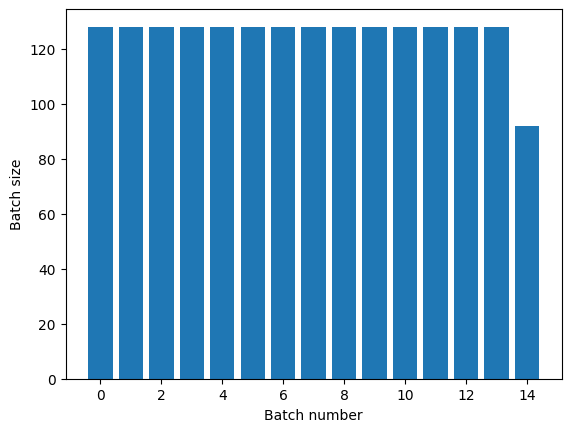If you need clear epoch separation, put `Dataset.batch` before the repeat:

``````titanic_batches = titanic_lines.batch(128).repeat(3)

plot_batch_sizes(titanic_batches)
``````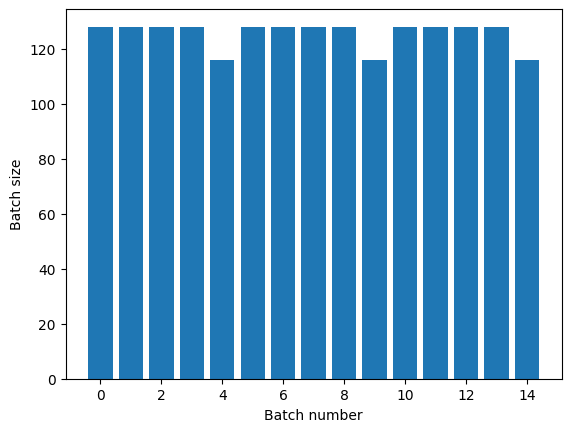If you would like to perform a custom computation (for example, to collect statistics) at the end of each epoch then it's simplest to restart the dataset iteration on each epoch:

``````epochs = 3
dataset = titanic_lines.batch(128)

for epoch in range(epochs):
for batch in dataset:
print(batch.shape)
print("End of epoch: ", epoch)
``````
```(128,)
(128,)
(128,)
(128,)
(116,)
End of epoch:  0
(128,)
(128,)
(128,)
(128,)
(116,)
End of epoch:  1
(128,)
(128,)
(128,)
(128,)
(116,)
End of epoch:  2
```

### Randomly shuffling input data

The `Dataset.shuffle()` transformation maintains a fixed-size buffer and chooses the next element uniformly at random from that buffer.

Add an index to the dataset so you can see the effect:

``````lines = tf.data.TextLineDataset(titanic_file)
counter = tf.data.experimental.Counter()

dataset = tf.data.Dataset.zip((counter, lines))
dataset = dataset.shuffle(buffer_size=100)
dataset = dataset.batch(20)
dataset
``````
```WARNING:tensorflow:From /tmpfs/tmp/ipykernel_13415/4092668703.py:2: CounterV2 (from tensorflow.python.data.experimental.ops.counter) is deprecated and will be removed in a future version.
Instructions for updating:
<BatchDataset element_spec=(TensorSpec(shape=(None,), dtype=tf.int64, name=None), TensorSpec(shape=(None,), dtype=tf.string, name=None))>
```

Since the `buffer_size` is 100, and the batch size is 20, the first batch contains no elements with an index over 120.

``````n,line_batch = next(iter(dataset))
print(n.numpy())
``````
```[ 65  58  36   5  52  31  81  19   3  53   4  72  11  97  22  25  50  80
92 118]
```

As with `Dataset.batch` the order relative to `Dataset.repeat` matters.

`Dataset.shuffle` doesn't signal the end of an epoch until the shuffle buffer is empty. So a shuffle placed before a repeat will show every element of one epoch before moving to the next:

``````dataset = tf.data.Dataset.zip((counter, lines))
shuffled = dataset.shuffle(buffer_size=100).batch(10).repeat(2)

print("Here are the item ID's near the epoch boundary:\n")
for n, line_batch in shuffled.skip(60).take(5):
print(n.numpy())
``````
```Here are the item ID's near the epoch boundary:

[618 447  44 620 475 474 477 573 594 600]
[504 580 577 578 569 611 551 571 459  76]
[507 536 581 599 534 513 590 604]
[ 95  99  44  55 101 103  16 106  64   3]
[ 28  46  72   7   5 104  21  83   2  91]
```
``````shuffle_repeat = [n.numpy().mean() for n, line_batch in shuffled]
plt.plot(shuffle_repeat, label="shuffle().repeat()")
plt.ylabel("Mean item ID")
plt.legend()
``````
```<matplotlib.legend.Legend at 0x7f0b3fc0ecd0>
```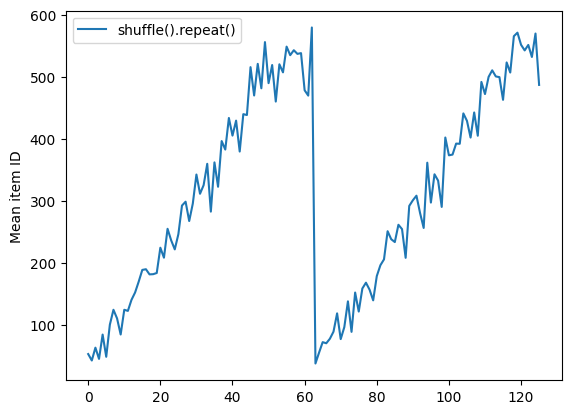But a repeat before a shuffle mixes the epoch boundaries together:

``````dataset = tf.data.Dataset.zip((counter, lines))
shuffled = dataset.repeat(2).shuffle(buffer_size=100).batch(10)

print("Here are the item ID's near the epoch boundary:\n")
for n, line_batch in shuffled.skip(55).take(15):
print(n.numpy())
``````
```Here are the item ID's near the epoch boundary:

[434 608 499 550 466 479 443   5 557 604]
[464  26  33 530 481 583   7 482 490 575]
[526   6 597 500 520 454 473 555 560 603]
[529 586 546 606  50  28 533 548 547  57]
[389  44  35 581   8 623 590  19  48  15]
[ 25   3 491  47 503 578  12  40  39  42]
[609 580 614  11 477  23  55  14  32 611]
[622  85  93 527  75  20  60 596  66  89]
[ 51  36  92  24  97 105 616 415  64  58]
[ 84 504  17 494   1 110 615  98 576 111]
[601  27 574 624  77 116  49 124 117  76]
[ 81 112  38 104  69   9 126  31 138 119]
[ 52 487 137  99 125  96 118 460  65 121]
[585 584 431 617  61  46 122  78 618 106]
[ 80  90 128  71  63 626 157 577 129 627]
```
``````repeat_shuffle = [n.numpy().mean() for n, line_batch in shuffled]

plt.plot(shuffle_repeat, label="shuffle().repeat()")
plt.plot(repeat_shuffle, label="repeat().shuffle()")
plt.ylabel("Mean item ID")
plt.legend()
``````
```<matplotlib.legend.Legend at 0x7f0b3fb6ddc0>
```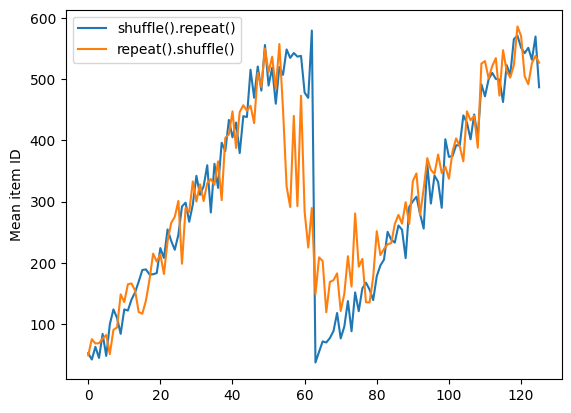## Preprocessing data

The `Dataset.map(f)` transformation produces a new dataset by applying a given function `f` to each element of the input dataset. It is based on the `map()` function that is commonly applied to lists (and other structures) in functional programming languages. The function `f` takes the `tf.Tensor` objects that represent a single element in the input, and returns the `tf.Tensor` objects that will represent a single element in the new dataset. Its implementation uses standard TensorFlow operations to transform one element into another.

This section covers common examples of how to use `Dataset.map()`.

### Decoding image data and resizing it

When training a neural network on real-world image data, it is often necessary to convert images of different sizes to a common size, so that they may be batched into a fixed size.

Rebuild the flower filenames dataset:

``````list_ds = tf.data.Dataset.list_files(str(flowers_root/'*/*'))
``````

Write a function that manipulates the dataset elements.

``````# Reads an image from a file, decodes it into a dense tensor, and resizes it
# to a fixed shape.
def parse_image(filename):
parts = tf.strings.split(filename, os.sep)
label = parts[-2]

image = tf.io.decode_jpeg(image)
image = tf.image.convert_image_dtype(image, tf.float32)
image = tf.image.resize(image, [128, 128])
return image, label
``````

Test that it works.

``````file_path = next(iter(list_ds))
image, label = parse_image(file_path)

def show(image, label):
plt.figure()
plt.imshow(image)
plt.title(label.numpy().decode('utf-8'))
plt.axis('off')

show(image, label)
``````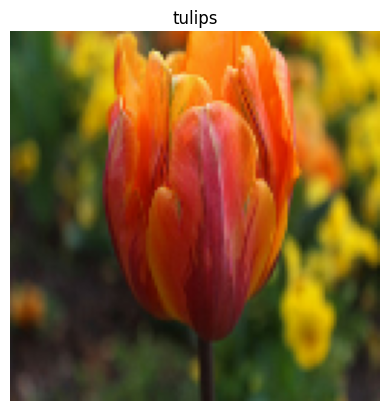Map it over the dataset.

``````images_ds = list_ds.map(parse_image)

for image, label in images_ds.take(2):
show(image, label)
``````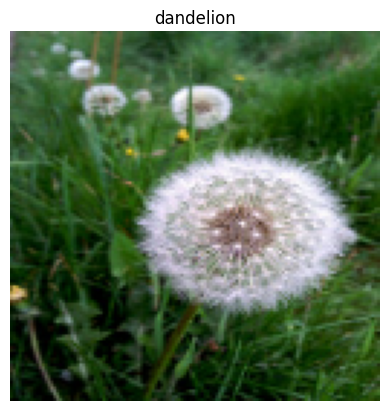### Applying arbitrary Python logic

For performance reasons, use TensorFlow operations for preprocessing your data whenever possible. However, it is sometimes useful to call external Python libraries when parsing your input data. You can use the `tf.py_function` operation in a `Dataset.map` transformation.

For example, if you want to apply a random rotation, the `tf.image` module only has `tf.image.rot90`, which is not very useful for image augmentation.

To demonstrate `tf.py_function`, try using the `scipy.ndimage.rotate` function instead:

``````import scipy.ndimage as ndimage

def random_rotate_image(image):
image = ndimage.rotate(image, np.random.uniform(-30, 30), reshape=False)
return image
``````
``````image, label = next(iter(images_ds))
image = random_rotate_image(image)
show(image, label)
``````
```Clipping input data to the valid range for imshow with RGB data ([0..1] for floats or [0..255] for integers).
```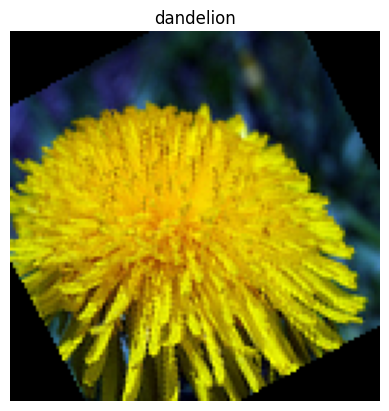To use this function with `Dataset.map` the same caveats apply as with `Dataset.from_generator`, you need to describe the return shapes and types when you apply the function:

``````def tf_random_rotate_image(image, label):
im_shape = image.shape
[image,] = tf.py_function(random_rotate_image, [image], [tf.float32])
image.set_shape(im_shape)
return image, label
``````
``````rot_ds = images_ds.map(tf_random_rotate_image)

for image, label in rot_ds.take(2):
show(image, label)
``````
```Clipping input data to the valid range for imshow with RGB data ([0..1] for floats or [0..255] for integers).
Clipping input data to the valid range for imshow with RGB data ([0..1] for floats or [0..255] for integers).
```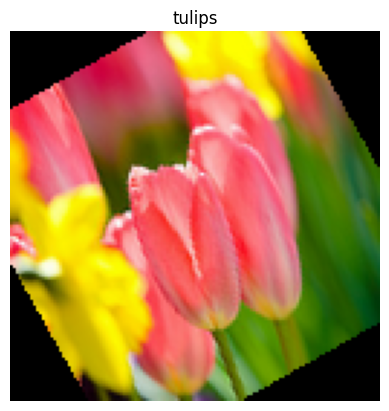### Parsing `tf.Example` protocol buffer messages

Many input pipelines extract `tf.train.Example` protocol buffer messages from a TFRecord format. Each `tf.train.Example` record contains one or more "features", and the input pipeline typically converts these features into tensors.

``````fsns_test_file = tf.keras.utils.get_file("fsns.tfrec", "https://storage.googleapis.com/download.tensorflow.org/data/fsns-20160927/testdata/fsns-00000-of-00001")
dataset = tf.data.TFRecordDataset(filenames = [fsns_test_file])
dataset
``````
```<TFRecordDatasetV2 element_spec=TensorSpec(shape=(), dtype=tf.string, name=None)>
```

You can work with `tf.train.Example` protos outside of a `tf.data.Dataset` to understand the data:

``````raw_example = next(iter(dataset))
parsed = tf.train.Example.FromString(raw_example.numpy())

feature = parsed.features.feature
raw_img = feature['image/encoded'].bytes_list.value
img = tf.image.decode_png(raw_img)
plt.imshow(img)
plt.axis('off')
_ = plt.title(feature["image/text"].bytes_list.value)
````````````raw_example = next(iter(dataset))
``````
``````def tf_parse(eg):
example = tf.io.parse_example(
eg[tf.newaxis], {
'image/encoded': tf.io.FixedLenFeature(shape=(), dtype=tf.string),
'image/text': tf.io.FixedLenFeature(shape=(), dtype=tf.string)
})
return example['image/encoded'], example['image/text']
``````
``````img, txt = tf_parse(raw_example)
print(txt.numpy())
print(repr(img.numpy()[:20]), "...")
``````
```b'Rue Perreyon'
b'\x89PNG\r\n\x1a\n\x00\x00\x00\rIHDR\x00\x00\x02X' ...
```
``````decoded = dataset.map(tf_parse)
decoded
``````
```<MapDataset element_spec=(TensorSpec(shape=(), dtype=tf.string, name=None), TensorSpec(shape=(), dtype=tf.string, name=None))>
```
``````image_batch, text_batch = next(iter(decoded.batch(10)))
image_batch.shape
``````
```TensorShape()
```

### Time series windowing

For an end-to-end time series example see: Time series forecasting.

Time series data is often organized with the time axis intact.

Use a simple `Dataset.range` to demonstrate:

``````range_ds = tf.data.Dataset.range(100000)
``````

Typically, models based on this sort of data will want a contiguous time slice.

The simplest approach would be to batch the data:

#### Using `batch`

``````batches = range_ds.batch(10, drop_remainder=True)

for batch in batches.take(5):
print(batch.numpy())
``````
```[0 1 2 3 4 5 6 7 8 9]
[10 11 12 13 14 15 16 17 18 19]
[20 21 22 23 24 25 26 27 28 29]
[30 31 32 33 34 35 36 37 38 39]
[40 41 42 43 44 45 46 47 48 49]
```

Or to make dense predictions one step into the future, you might shift the features and labels by one step relative to each other:

``````def dense_1_step(batch):
# Shift features and labels one step relative to each other.
return batch[:-1], batch[1:]

predict_dense_1_step = batches.map(dense_1_step)

for features, label in predict_dense_1_step.take(3):
print(features.numpy(), " => ", label.numpy())
``````
```[0 1 2 3 4 5 6 7 8]  =>  [1 2 3 4 5 6 7 8 9]
[10 11 12 13 14 15 16 17 18]  =>  [11 12 13 14 15 16 17 18 19]
[20 21 22 23 24 25 26 27 28]  =>  [21 22 23 24 25 26 27 28 29]
```

To predict a whole window instead of a fixed offset you can split the batches into two parts:

``````batches = range_ds.batch(15, drop_remainder=True)

def label_next_5_steps(batch):
return (batch[:-5],   # Inputs: All except the last 5 steps
batch[-5:])   # Labels: The last 5 steps

predict_5_steps = batches.map(label_next_5_steps)

for features, label in predict_5_steps.take(3):
print(features.numpy(), " => ", label.numpy())
``````
```[0 1 2 3 4 5 6 7 8 9]  =>  [10 11 12 13 14]
[15 16 17 18 19 20 21 22 23 24]  =>  [25 26 27 28 29]
[30 31 32 33 34 35 36 37 38 39]  =>  [40 41 42 43 44]
```

To allow some overlap between the features of one batch and the labels of another, use `Dataset.zip`:

``````feature_length = 10
label_length = 3

features = range_ds.batch(feature_length, drop_remainder=True)
labels = range_ds.batch(feature_length).skip(1).map(lambda labels: labels[:label_length])

predicted_steps = tf.data.Dataset.zip((features, labels))

for features, label in predicted_steps.take(5):
print(features.numpy(), " => ", label.numpy())
``````
```[0 1 2 3 4 5 6 7 8 9]  =>  [10 11 12]
[10 11 12 13 14 15 16 17 18 19]  =>  [20 21 22]
[20 21 22 23 24 25 26 27 28 29]  =>  [30 31 32]
[30 31 32 33 34 35 36 37 38 39]  =>  [40 41 42]
[40 41 42 43 44 45 46 47 48 49]  =>  [50 51 52]
```

#### Using `window`

While using `Dataset.batch` works, there are situations where you may need finer control. The `Dataset.window` method gives you complete control, but requires some care: it returns a `Dataset` of `Datasets`. Go to the Dataset structure section for details.

``````window_size = 5

windows = range_ds.window(window_size, shift=1)
for sub_ds in windows.take(5):
print(sub_ds)
``````
```<_VariantDataset element_spec=TensorSpec(shape=(), dtype=tf.int64, name=None)>
<_VariantDataset element_spec=TensorSpec(shape=(), dtype=tf.int64, name=None)>
<_VariantDataset element_spec=TensorSpec(shape=(), dtype=tf.int64, name=None)>
<_VariantDataset element_spec=TensorSpec(shape=(), dtype=tf.int64, name=None)>
<_VariantDataset element_spec=TensorSpec(shape=(), dtype=tf.int64, name=None)>
```

The `Dataset.flat_map` method can take a dataset of datasets and flatten it into a single dataset:

``````for x in windows.flat_map(lambda x: x).take(30):
print(x.numpy(), end=' ')
``````
```0 1 2 3 4 1 2 3 4 5 2 3 4 5 6 3 4 5 6 7 4 5 6 7 8 5 6 7 8 9
```

In nearly all cases, you will want to `Dataset.batch` the dataset first:

``````def sub_to_batch(sub):
return sub.batch(window_size, drop_remainder=True)

for example in windows.flat_map(sub_to_batch).take(5):
print(example.numpy())
``````
```[0 1 2 3 4]
[1 2 3 4 5]
[2 3 4 5 6]
[3 4 5 6 7]
[4 5 6 7 8]
```

Now, you can see that the `shift` argument controls how much each window moves over.

Putting this together you might write this function:

``````def make_window_dataset(ds, window_size=5, shift=1, stride=1):
windows = ds.window(window_size, shift=shift, stride=stride)

def sub_to_batch(sub):
return sub.batch(window_size, drop_remainder=True)

windows = windows.flat_map(sub_to_batch)
return windows
``````
``````ds = make_window_dataset(range_ds, window_size=10, shift = 5, stride=3)

for example in ds.take(10):
print(example.numpy())
``````
```[ 0  3  6  9 12 15 18 21 24 27]
[ 5  8 11 14 17 20 23 26 29 32]
[10 13 16 19 22 25 28 31 34 37]
[15 18 21 24 27 30 33 36 39 42]
[20 23 26 29 32 35 38 41 44 47]
[25 28 31 34 37 40 43 46 49 52]
[30 33 36 39 42 45 48 51 54 57]
[35 38 41 44 47 50 53 56 59 62]
[40 43 46 49 52 55 58 61 64 67]
[45 48 51 54 57 60 63 66 69 72]
```

Then it's easy to extract labels, as before:

``````dense_labels_ds = ds.map(dense_1_step)

for inputs,labels in dense_labels_ds.take(3):
print(inputs.numpy(), "=>", labels.numpy())
``````
```[ 0  3  6  9 12 15 18 21 24] => [ 3  6  9 12 15 18 21 24 27]
[ 5  8 11 14 17 20 23 26 29] => [ 8 11 14 17 20 23 26 29 32]
[10 13 16 19 22 25 28 31 34] => [13 16 19 22 25 28 31 34 37]
```

### Resampling

When working with a dataset that is very class-imbalanced, you may want to resample the dataset. `tf.data` provides two methods to do this. The credit card fraud dataset is a good example of this sort of problem.

``````zip_path = tf.keras.utils.get_file(
fname='creditcard.zip',
extract=True)

csv_path = zip_path.replace('.zip', '.csv')
``````
```Downloading data from https://storage.googleapis.com/download.tensorflow.org/data/creditcard.zip
69155632/69155632 [==============================] - 0s 0us/step
```
``````creditcard_ds = tf.data.experimental.make_csv_dataset(
csv_path, batch_size=1024, label_name="Class",
# Set the column types: 30 floats and an int.
column_defaults=[float()]*30+[int()])
``````

Now, check the distribution of classes, it is highly skewed:

``````def count(counts, batch):
features, labels = batch
class_1 = labels == 1
class_1 = tf.cast(class_1, tf.int32)

class_0 = labels == 0
class_0 = tf.cast(class_0, tf.int32)

counts['class_0'] += tf.reduce_sum(class_0)
counts['class_1'] += tf.reduce_sum(class_1)

return counts
``````
``````counts = creditcard_ds.take(10).reduce(
initial_state={'class_0': 0, 'class_1': 0},
reduce_func = count)

counts = np.array([counts['class_0'].numpy(),
counts['class_1'].numpy()]).astype(np.float32)

fractions = counts/counts.sum()
print(fractions)
``````
```[0.9965 0.0035]
```

A common approach to training with an imbalanced dataset is to balance it. `tf.data` includes a few methods which enable this workflow:

#### Datasets sampling

One approach to resampling a dataset is to use `sample_from_datasets`. This is more applicable when you have a separate `tf.data.Dataset` for each class.

Here, just use filter to generate them from the credit card fraud data:

``````negative_ds = (
creditcard_ds
.unbatch()
.filter(lambda features, label: label==0)
.repeat())
positive_ds = (
creditcard_ds
.unbatch()
.filter(lambda features, label: label==1)
.repeat())
``````
``````for features, label in positive_ds.batch(10).take(1):
print(label.numpy())
``````
```[1 1 1 1 1 1 1 1 1 1]
```

To use `tf.data.Dataset.sample_from_datasets` pass the datasets, and the weight for each:

``````balanced_ds = tf.data.Dataset.sample_from_datasets(
[negative_ds, positive_ds], [0.5, 0.5]).batch(10)
``````

Now the dataset produces examples of each class with a 50/50 probability:

``````for features, labels in balanced_ds.take(10):
print(labels.numpy())
``````
```[1 0 0 0 1 0 0 0 0 1]
[0 1 1 0 0 0 1 1 1 0]
[0 1 1 0 1 1 1 1 1 1]
[1 0 1 1 0 1 1 1 1 0]
[0 0 0 1 0 1 0 1 0 0]
[1 0 1 0 1 1 1 1 1 1]
[0 1 1 1 1 0 1 0 0 1]
[1 1 0 0 1 0 1 1 0 1]
[0 1 1 0 0 0 0 1 0 0]
[0 0 0 1 1 0 1 1 0 0]
```

#### Rejection resampling

One problem with the above `Dataset.sample_from_datasets` approach is that it needs a separate `tf.data.Dataset` per class. You could use `Dataset.filter` to create those two datasets, but that results in all the data being loaded twice.

The `tf.data.Dataset.rejection_resample` method can be applied to a dataset to rebalance it, while only loading it once. Elements will be dropped or repeated to achieve balance.

The `rejection_resample` method takes a `class_func` argument. This `class_func` is applied to each dataset element, and is used to determine which class an example belongs to for the purposes of balancing.

The goal here is to balance the label distribution, and the elements of `creditcard_ds` are already `(features, label)` pairs. So the `class_func` just needs to return those labels:

``````def class_func(features, label):
return label
``````

The resampling method deals with individual examples, so in this case you must `unbatch` the dataset before applying that method.

The method needs a target distribution, and optionally an initial distribution estimate as inputs.

``````resample_ds = (
creditcard_ds
.unbatch()
.rejection_resample(class_func, target_dist=[0.5,0.5],
initial_dist=fractions)
.batch(10))
``````
```WARNING:tensorflow:From /tmpfs/src/tf_docs_env/lib/python3.9/site-packages/tensorflow/python/data/ops/dataset_ops.py:6041: Print (from tensorflow.python.ops.logging_ops) is deprecated and will be removed after 2018-08-20.
Instructions for updating:
Use tf.print instead of tf.Print. Note that tf.print returns a no-output operator that directly prints the output. Outside of defuns or eager mode, this operator will not be executed unless it is directly specified in session.run or used as a control dependency for other operators. This is only a concern in graph mode. Below is an example of how to ensure tf.print executes in graph mode:
```

The `rejection_resample` method returns `(class, example)` pairs where the `class` is the output of the `class_func`. In this case, the `example` was already a `(feature, label)` pair, so use `map` to drop the extra copy of the labels:

``````balanced_ds = resample_ds.map(lambda extra_label, features_and_label: features_and_label)
``````

Now the dataset produces examples of each class with a 50/50 probability:

``````for features, labels in balanced_ds.take(10):
print(labels.numpy())
``````
```Proportion of examples rejected by sampler is high: [0.996484399][0.996484399 0.00351562491][0 1]
Proportion of examples rejected by sampler is high: [0.996484399][0.996484399 0.00351562491][0 1]
Proportion of examples rejected by sampler is high: [0.996484399][0.996484399 0.00351562491][0 1]
Proportion of examples rejected by sampler is high: [0.996484399][0.996484399 0.00351562491][0 1]
Proportion of examples rejected by sampler is high: [0.996484399][0.996484399 0.00351562491][0 1]
Proportion of examples rejected by sampler is high: [0.996484399][0.996484399 0.00351562491][0 1]
Proportion of examples rejected by sampler is high: [0.996484399][0.996484399 0.00351562491][0 1]
Proportion of examples rejected by sampler is high: [0.996484399][0.996484399 0.00351562491][0 1]
Proportion of examples rejected by sampler is high: [0.996484399][0.996484399 0.00351562491][0 1]
Proportion of examples rejected by sampler is high: [0.996484399][0.996484399 0.00351562491][0 1]
[1 1 1 0 0 0 1 0 0 0]
[1 0 0 1 0 1 1 0 1 1]
[1 1 0 0 0 0 1 0 1 1]
[0 1 0 1 0 0 0 1 0 1]
[0 1 0 1 0 0 1 1 0 0]
[0 1 0 0 0 1 0 1 0 0]
[0 1 0 0 0 0 1 1 0 0]
[1 0 1 0 0 0 1 1 0 0]
[1 1 1 0 1 1 1 1 1 0]
[0 0 1 0 1 1 1 0 1 0]
```

## Iterator Checkpointing

Tensorflow supports taking checkpoints so that when your training process restarts it can restore the latest checkpoint to recover most of its progress. In addition to checkpointing the model variables, you can also checkpoint the progress of the dataset iterator. This could be useful if you have a large dataset and don't want to start the dataset from the beginning on each restart. Note however that iterator checkpoints may be large, since transformations such as `Dataset.shuffle` and `Dataset.prefetch` require buffering elements within the iterator.

To include your iterator in a checkpoint, pass the iterator to the `tf.train.Checkpoint` constructor.

``````range_ds = tf.data.Dataset.range(20)

iterator = iter(range_ds)
ckpt = tf.train.Checkpoint(step=tf.Variable(0), iterator=iterator)
manager = tf.train.CheckpointManager(ckpt, '/tmp/my_ckpt', max_to_keep=3)

print([next(iterator).numpy() for _ in range(5)])

save_path = manager.save()

print([next(iterator).numpy() for _ in range(5)])

ckpt.restore(manager.latest_checkpoint)

print([next(iterator).numpy() for _ in range(5)])
``````
```[0, 1, 2, 3, 4]
[5, 6, 7, 8, 9]
[5, 6, 7, 8, 9]
```

## Using `tf.data` with `tf.keras`

The `tf.keras` API simplifies many aspects of creating and executing machine learning models. Its `Model.fit` and `Model.evaluate` and `Model.predict` APIs support datasets as inputs. Here is a quick dataset and model setup:

``````train, test = tf.keras.datasets.fashion_mnist.load_data()

images, labels = train
images = images/255.0
labels = labels.astype(np.int32)
``````
``````fmnist_train_ds = tf.data.Dataset.from_tensor_slices((images, labels))
fmnist_train_ds = fmnist_train_ds.shuffle(5000).batch(32)

model = tf.keras.Sequential([
tf.keras.layers.Flatten(),
tf.keras.layers.Dense(10)
])

loss=tf.keras.losses.SparseCategoricalCrossentropy(from_logits=True),
metrics=['accuracy'])
``````

Passing a dataset of `(feature, label)` pairs is all that's needed for `Model.fit` and `Model.evaluate`:

``````model.fit(fmnist_train_ds, epochs=2)
``````
```Epoch 1/2
1875/1875 [==============================] - 4s 2ms/step - loss: 0.5999 - accuracy: 0.7975
Epoch 2/2
1875/1875 [==============================] - 4s 2ms/step - loss: 0.4620 - accuracy: 0.8416
<keras.callbacks.History at 0x7f0b3f882ac0>
```

If you pass an infinite dataset, for example by calling `Dataset.repeat`, you just need to also pass the `steps_per_epoch` argument:

``````model.fit(fmnist_train_ds.repeat(), epochs=2, steps_per_epoch=20)
``````
```Epoch 1/2
20/20 [==============================] - 0s 2ms/step - loss: 0.5123 - accuracy: 0.8406
Epoch 2/2
20/20 [==============================] - 0s 2ms/step - loss: 0.3830 - accuracy: 0.8578
<keras.callbacks.History at 0x7f0b3fc8dd60>
```

For evaluation you can pass the number of evaluation steps:

``````loss, accuracy = model.evaluate(fmnist_train_ds)
print("Loss :", loss)
print("Accuracy :", accuracy)
``````
```1875/1875 [==============================] - 3s 2ms/step - loss: 0.4430 - accuracy: 0.8485
Loss : 0.4429807662963867
Accuracy : 0.848466694355011
```

For long datasets, set the number of steps to evaluate:

``````loss, accuracy = model.evaluate(fmnist_train_ds.repeat(), steps=10)
print("Loss :", loss)
print("Accuracy :", accuracy)
``````
```10/10 [==============================] - 0s 2ms/step - loss: 0.4909 - accuracy: 0.8313
Loss : 0.4908931851387024
Accuracy : 0.831250011920929
```

The labels are not required when calling `Model.predict`.

``````predict_ds = tf.data.Dataset.from_tensor_slices(images).batch(32)
result = model.predict(predict_ds, steps = 10)
print(result.shape)
``````
```10/10 [==============================] - 0s 1ms/step
(320, 10)
```

But the labels are ignored if you do pass a dataset containing them:

``````result = model.predict(fmnist_train_ds, steps = 10)
print(result.shape)
``````
```10/10 [==============================] - 0s 1ms/step
(320, 10)
```
[{ "type": "thumb-down", "id": "missingTheInformationINeed", "label":"Missing the information I need" },{ "type": "thumb-down", "id": "tooComplicatedTooManySteps", "label":"Too complicated / too many steps" },{ "type": "thumb-down", "id": "outOfDate", "label":"Out of date" },{ "type": "thumb-down", "id": "samplesCodeIssue", "label":"Samples / code issue" },{ "type": "thumb-down", "id": "otherDown", "label":"Other" }]
[{ "type": "thumb-up", "id": "easyToUnderstand", "label":"Easy to understand" },{ "type": "thumb-up", "id": "solvedMyProblem", "label":"Solved my problem" },{ "type": "thumb-up", "id": "otherUp", "label":"Other" }]